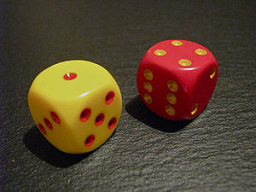# Probability 64634

What probability does a randomly drawn two-digit number have the same digits? Write the result as a decimal number.

p =  1/10

### Step-by-step explanation:Did you find an error or inaccuracy? Feel free to write us. Thank you!

Tips to related online calculators
Need help to calculate sum, simplify or multiply fractions? Try our fraction calculator.
Would you like to compute count of combinations?